Next: Keplerian orbits Up: Newtonian gravity Previous: Potential due to uniform

# Exercises

1. A particle is projected vertically upward from the Earth's surface with a velocity that would, were gravity uniform, carry it to a height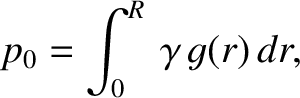. Show that if the variation of gravity with height is allowed for, but the resistance of air is neglected, then the height reached will be greater by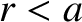, where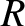is the Earth's radius. (From Lamb 1923.)

2. A particle is projected vertically upward from the Earth's surface with a velocity just sufficient for it to reach infinity (neglecting air resistance). Prove that the time needed to reach a heightis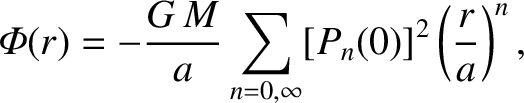whereis the Earth's radius, and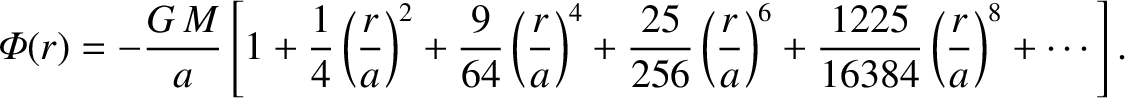its surface gravitational acceleration. (From Lamb 1923.)

3. Assuming that the Earth is a sphere of radius, and neglecting air resistance, show that a particle that starts from rest a distancefrom the Earth's surface will reach the surface with speed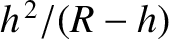after a time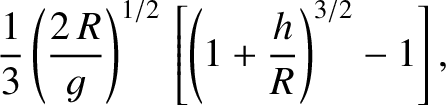, whereis the surface gravitational acceleration. (Modified from Smart 1951.)

4. Demonstrate that if a narrow shaft were drilled though the center of a uniform self-gravitating sphere then a test mass moving in this shaft executes simple harmonic motion about the center of the sphere with period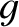whereis the radius of the sphere, andthe gravitational acceleration at its surface.

5. Consider an isolated system consisting of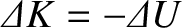point objects interacting via gravity. The equation of motion of the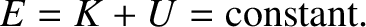th object is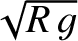where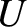and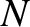are the mass and position vector of this object, respectively. Moreover, the total potential energy of the system takes the form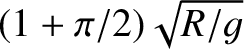Write an expression for the total kinetic energy,. Demonstrate, from the equations of motion, that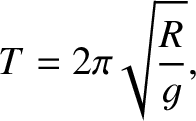is constant in time.

6. Consider a function of many variables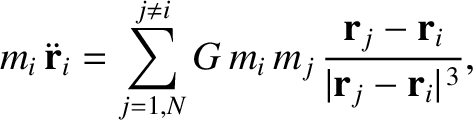. Such a function that satisfies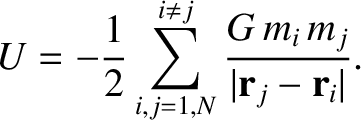for all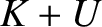, and all values of the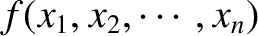, is termed a homogeneous function of degree. Prove the following theorem regarding homogeneous functions: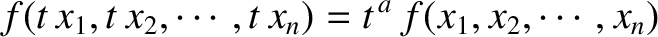7. Consider an isolated system consisting ofpoint particles interacting via attractive central forces. Let the mass and position vector of theth particle beand, respectively. Suppose that magnitude of the force exerted on particleby particle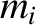is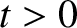. Here,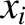measures some constant physical property of theth particle (e.g., its electric charge). Show that the total potential energy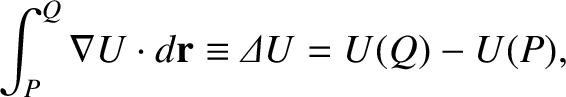of the system is written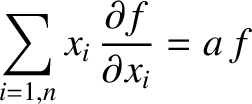Is this a homogeneous function? If so, what is its degree? Demonstrate that the equation of motion of theth particle can be written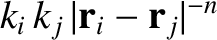(This is shorthand for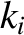,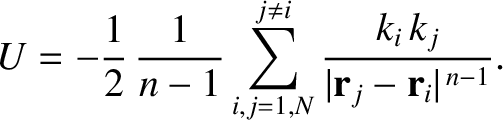, etc., where the,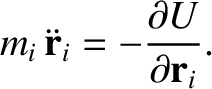,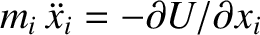, for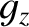, are treated as independent variables.) Use the mathematical theorem from the previous exercise to show that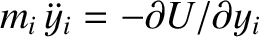where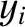, andis the total kinetic energy. This result is known as the virial theorem. Demonstrate that when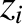the system possesses no virial equilibria (i.e., states for which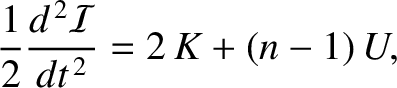does not evolve in time) that are bounded.

8. Demonstrate that the gravitational potential energy of a spherically symmetric mass distribution of mass density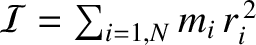that extends out to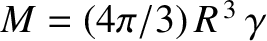can be written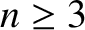Hence, show that if the mass distribution is such that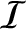where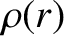, then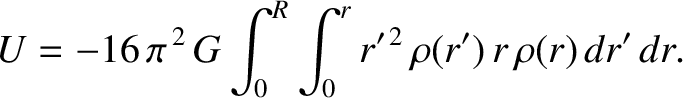where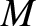is the total mass.

9. A globular star cluster can be approximated as an isolated self-gravitating virial equilibrium consisting of a great number of equal mass stars. Demonstrate, from the virial theorem, that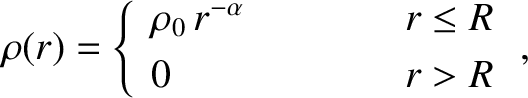for such a cluster. Suppose that the stars in a given cluster are uniformly distributed throughout a spherical volume. Show that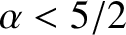where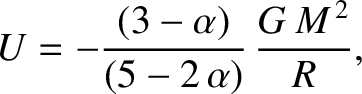is the mean stellar velocity, and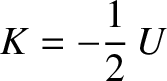is the escape speed (i.e., the speed a star at the edge of the cluster would require in order to escape to infinity.) See Exercise 7.

10. A star can be through of as a spherical system that consists of a very large number of particles, of massand position vector, interacting via gravity. Show that, for such a system, the virial theorem implies that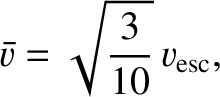where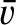is a constant,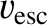, and the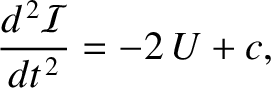are measured from the geometric center. Hence, deduce that the angular frequency of small-amplitude radial pulsations of the star (in which the radial displacement is directly proportional to the radial distance from the center) takes the form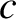where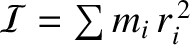and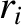are the equilibrium values ofand. Finally, show that if the mass density within the star varies as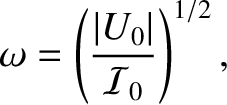, where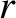is the radial distance from the geometric center, and where, then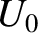whereandare the stellar mass and radius, respectively. See Exercises 7 and 8.Next: Keplerian orbits Up: Newtonian gravity Previous: Potential due to uniform
Richard Fitzpatrick 2016-03-31